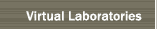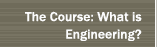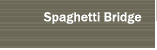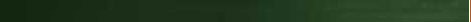Heat Conduction

Problem: Deduce the cross-sectional profile of a heated conductive bar based on its temperature.

Heat flows through thermally conductive materials by a process generally known as 'gradient transport'. Gradient heat transport depends on three quantities: the conductivity of the material, the cross-sectional area of the material, and the spatial gradient of temperature. The larger the conductivity, gradient,and/or cross-section, the faster the heat flows.

In this experiment, the flow of heat through a collection of conductive bars which vary in cross-section is simulated. The simulation is performed as a one-dimensional, time-dependent process--along the x-axis. Heat flow in the y-direction is assumed to be negligible, and variations in the cross-sections of the bars are taken into account by characterizing the conductivity C(x)of each bar a function of position. As the simulation begins, heat flows into the bar and changes the bar's temperature distribution. From that distribution, the task is to deduce the bar's cross-section.

Heat is applied to the bar at x=0 in one of three ways: as constant temperature, as constant heat flux, or as sinusoidal temperature. Heat is managed at the far end of the bar x=L in one of two ways: no heat loss, as constant temperature. Expressed as boundary conditions for temperature as a function of position and time: T(0,t)=100, dT(0,t)/dx=D, T(0,t)=A+Bcos(t), dT(L,t)/dx=0, T(L,t)=0.

There are two modes for running the experiment: 1) one in which temperature is recorded from movable temperature probes, and 2) one in which the temperature profile along the length of the bar is displayed. In both of these modes, boundary conditions and bar cross-sections are chosen randomly.

There is a third mode for the simulation: a practice mode in which the user can choose his own bar cross-sections and boundary conditions. In this case the simulation generates temperature profiles.

Try your luck at deducing the randomly-chosen bar in each of the first two modes. Click on 'done' when you're finished, and record the values of 'parameter'--the answer key. (Of course, try the practice mode first to get a sense for the experiment.)

Start the conduction experiment.